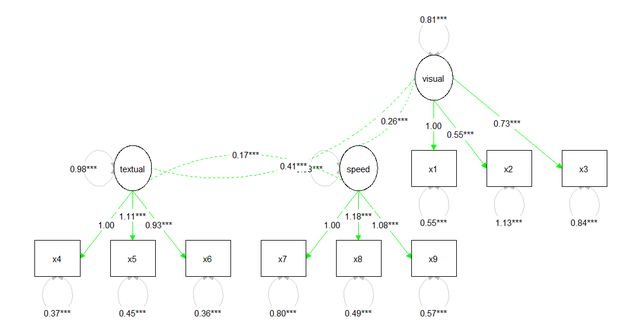# 例子说明

• 画图之前我们需要有一个画图的对象fit，也就是你的拟合成功的SEM模型
• 第二步是要将这个对象的图中的可以编辑的节点通过get_nodes(fit)得到，或者通过get_edges(fit)得到可以编辑的边
• 然后用get_layout()设定你想要的个性化布局
• 第四步就是用graph_sem出图了

# 先跑个CFA试试手？

``````library(lavaan)
HS.model <- ' visual  =~ x1 + x2 + x3
textual =~ x4 + x5 + x6
speed   =~ x7 + x8 + x9 '
fit <- cfa(HS.model, data=HolzingerSwineford1939)``````

``graph_sem(model = fit)``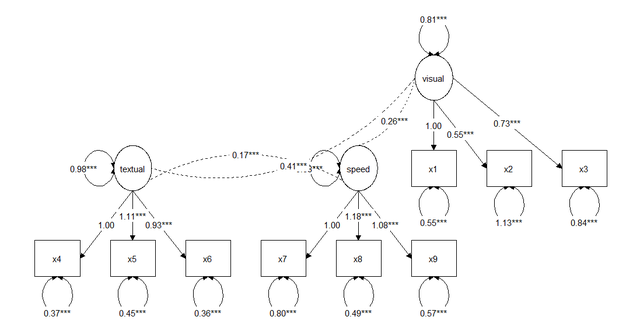``get_layout(fit)``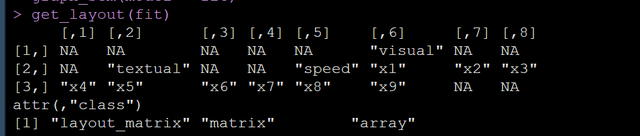``````lay <- get_layout( NA, "textual", NA,  NA,  "speed", NA,  NA, "visual", NA,
NA, NA,   NA,   NA, NA,   NA, NA, NA,   NA,
"x1",   "x2", "x3","x4", "x5",   "x6", "x7", "x8", "x9",rows = 3 )``````

``graph_sem(fit, layout = lay)``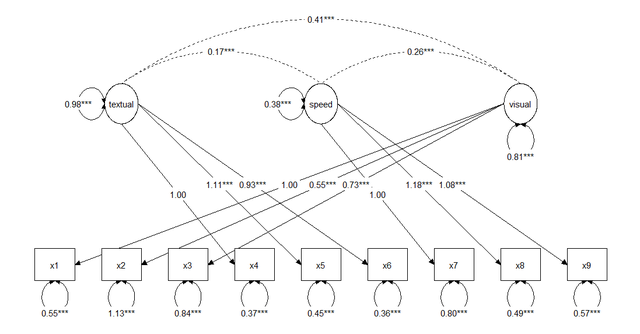# 自定义节点和边

``get_nodes(fit)``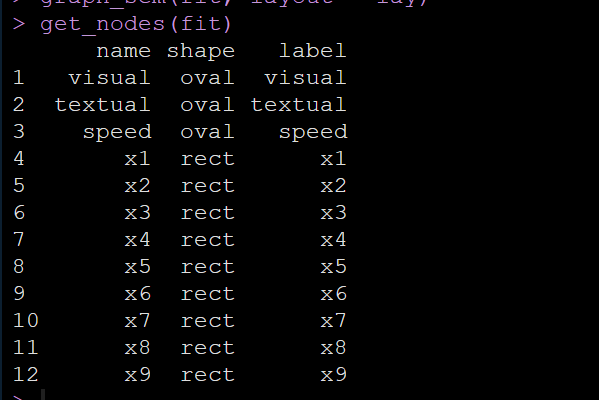``get_edges(fit)``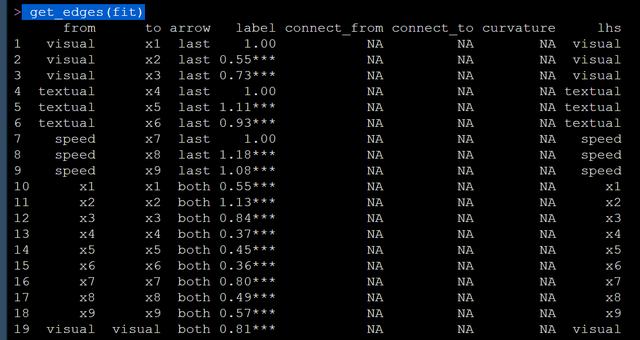``````prepare_graph(fit) %>%
edit_graph({ label = paste(p[,3]," Codewar") }) %>%
plot()``````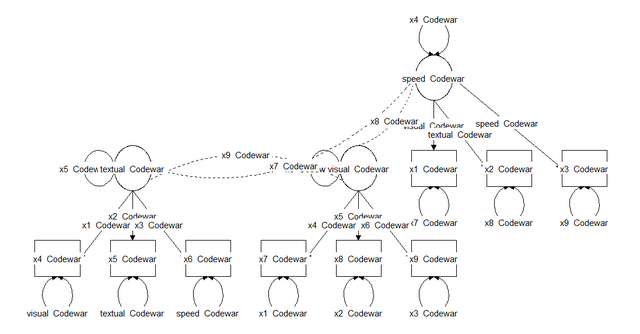``````prepare_graph(fit) %>%
edit_graph({ label_color = "blue" },element='nodes') %>%
plot()``````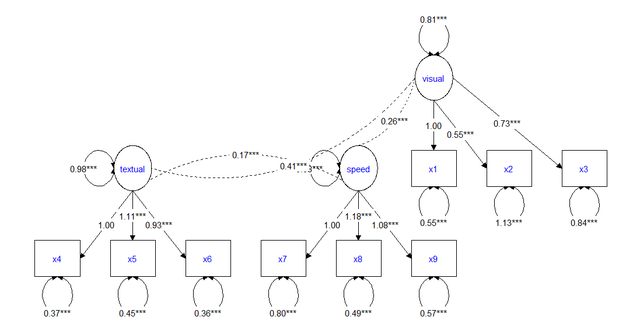• linetype: The type of line, e.g., continuous (linetype = 1 or dashed linetype = 2)
• colour or color: The color of the line (e.g., colour = "red" or colour = "blue")
• size: The size of the line (e.g., size = 1 or size = 2)
• alpha: The transparency of the line (e.g., alpha = 1 or alpha = .2)

• linetype: The type of line surrounding the node, e.g., continuous (linetype = 1 or dashed linetype = 2)
• colour or color: The color of the line surrounding the node (e.g., colour = "red" or colour = "blue")
• fill: The interior color of the node (e.g., colour = "red" or colour = "blue")
• size: The size of the line (e.g., size = 1 or size = 2)
• alpha: The transparency of the node (e.g., alpha = 1 or alpha = .2)

``````edges(graph_data) %>%
mutate(colour = "black") %>%
mutate(colour = replace(colour, from == "visual" & to == "x2", "red")) %>%
mutate(linetype = 1) %>%
mutate(linetype = replace(linetype, from == "visual" & to == "x2", 2)) %>%
mutate(alpha = 1) %>%
mutate(alpha = replace(alpha, from == "visual" & to == "x2", .5)) -> edges(graph_data)
plot(graph_data)``````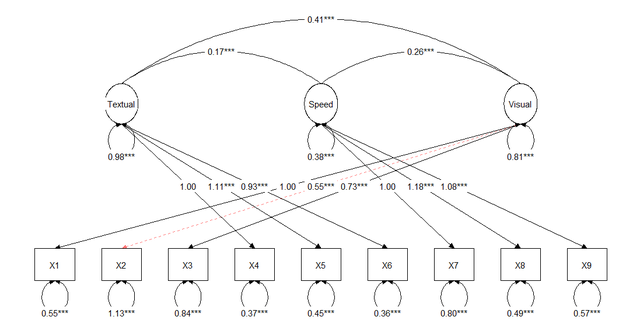``````prepare_graph(fit) %>%
color_pos_edges("green") %>%
color_neg_edges("red") %>%
color_var("black") %>%
alpha_var(.2) %>%
plot()``````